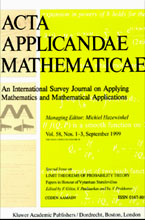## Symmetries of Partial Differential Equations

### Part I

Edited by: A. M. Vinogradov, Moscow State University (Russia)
Special Issue of Acta Applicandae Mathematicae, Volume 15, Issue 1/2, 1989, 210 pages, ISSN: 0167-8019.

Contents
• A. M. Vinogradov, Symmetries and conservation laws of partial differential equations: basic notions and results (pp. 3--21);
• V. N. Gusyatnikova, A. V. Samokhin, V. S. Titov, A. M. Vinogradov and V. A. Yumaguzhin, Symmetries and conservation laws of Kadomtsev-Pogutse equations (their computation and first applications) (pp. 23--64);
• V. N. Gusyatnikova and V. A. Yumaguzhin, Symmetries and conservation laws of Navier-Stokes equations (pp. 65--81);
• N. O. Sharomet, Symmetries, invariant solutions and conservation laws of the nonlinear acoustics equation (pp. 83--120);
• A. M. Verbovetsky, Local nonintegrability of long-short wave interaction equations (pp. 121--136);
• V. S. Titov, On symmetries and conservation laws of the equations of shallow water with an axisymmetric profile of bottom (pp. 137--147);
• Yu. R. Romanovsky, On symmetries of the heat equation (pp. 149--160);
• I. S. Krasilshchik and A. M. Vinogradov, Nonlocal trends in the geometry of differential equations: symmetries, conservation laws, and Backlund transformations (pp. 161--209).